# Decision tree makes flat prediction.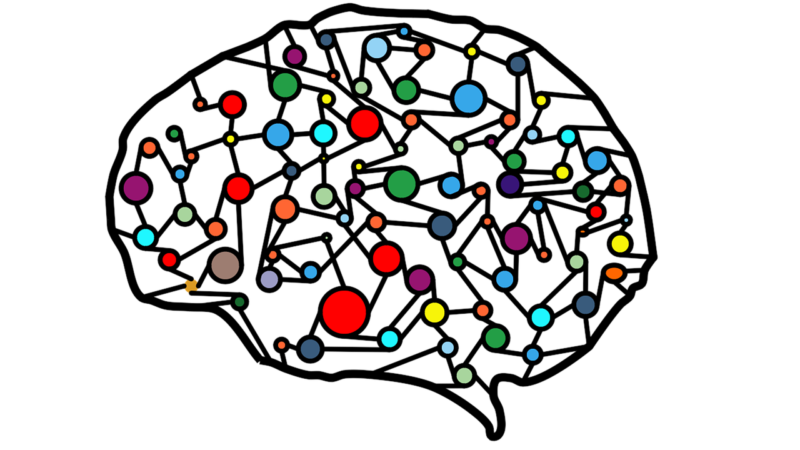Decision tree makes flat prediction.
When you use decision tree model like RandomForest or XGBoost or LightGBM, sometimes it makes flat result.

## Problem

If we prepare particular condition, decision tree always makes flat result.

### Decision tree always makes flat result

First we prepare increasing data like `y=0.5x` .

```import matplotlib.pyplot as plt
import numpy as np
from sklearn.model_selection import train_test_split
from sklearn.ensemble import RandomForestRegressor

# dataset
x = np.linspace(0, 10, 100)
y = x * 0.5

# Set data on graph
plt.plot(x, y, label="y=0.5x")
# Set legend
plt.legend()
# Show graph
plt.show()
```

Once data is prepared, it shows linear graph like below.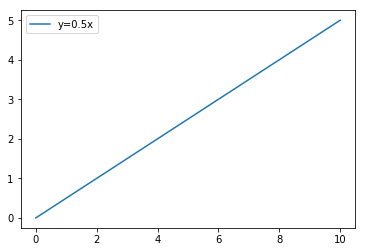We split this data into 70% training data and remaining test data.

```# split data
train_rate = 0.7
train_size = int(len(x) * train_rate)
x_train, x_test, y_train, y_test = x[0:train_size], x[train_size:len(x)], y[0:train_size], y[train_size:len(y)]

# Show graph
plt.plot(x_train, y_train, label="y=0.5x(train)")
plt.plot(x_test, y_test, label="y=0.5x(test)")
plt.legend()
plt.show()
```

Then linear data is separated into training data and test data.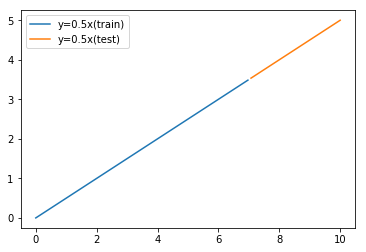And we train model with trainig data and predict with test data.
We use a kind of Decision tree model `RandomForest`.

```# Train and Predict
reg = RandomForestRegressor(random_state=0)
reg = reg.fit(np.array(x_train).reshape(-1,1), y_train)
y_pred = reg.predict(np.array(x_test).reshape(-1,1))

# Show graph
plt.plot(x_train, y_train, label="y=0.5x(train)")
plt.plot(x_test, y_test, label="y=0.5x(test)")
plt.plot(x_test, y_pred, label="y_pred")
plt.legend()
plt.show()
```

Then we get result like below.
Prediction result shows flat. It is different from actual test data.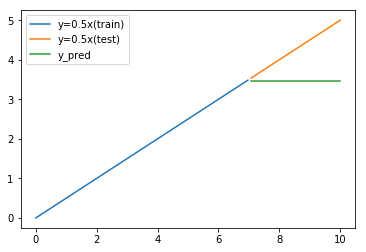### Comparing to Linear Regression

If we use Linear Regression model, it does not show flat result.

```# Train and Predict
from sklearn.linear_model import LinearRegression
reg = LinearRegression()
reg = reg.fit(np.array(x_train).reshape(-1,1), y_train)
y_pred = reg.predict(np.array(x_test).reshape(-1,1))

# Show graph
plt.plot(x_train, y_train, label="y=0.5x(train)")
plt.plot(x_test, y_test, label="y=0.5x(test)")
plt.plot(x_test, y_pred, label="y_pred")
plt.legend()
plt.show()
```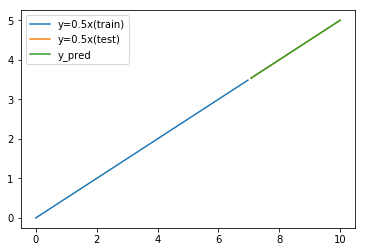## Reason why predicted data become flat

Reason why predicted data become flat is architecture of Decision tree.

Rule of Decision tree is below.
RandomForest and XGBoost are little bit more compricated.
But base of the architecture is same.

• Check input value and choose branch.
• End of branch has prediction result.
• Threshold values and edge values are fixed by training data.

Important thing is "edge values are fixed by training data".
Edge values are prediction values.
They are fixed in training phase.
After training, even we input bigger data, decision route and result is always maximum ome.

In last example of `y=0.5x`, maximum value of training data is `3.5` in case of `x=7`.
So trained model can't predict more than `3.5`.

It means Decision tree can't predict different range from training data.

## How to solve

So is Decision tree useless ?
No, it is not correct.

It can't predict unlimited range value.
So we can use limited range value as prediction target.

### Use difference

Assume x is separated value and use difference between y of t and t-1.
So we can get stable difference for the example of `y=0.5x`.

```import pandas as pd
df_data = pd.DataFrame()
df_data["x"] = x
df_data["y"] = y
df_data["y_diff"] = df_data["y"] - df_data["y"].shift(1)
df_data = df_data.dropna()

# split data
df_train, df_test = df_data.iloc[0:train_size], df_data.iloc[train_size:]

# Show graph
plt.plot(df_train["x"], df_train["y_diff"], label="y=0.5x(train) diff")
plt.plot(df_test["x"], df_test["y_diff"], label="y=0.5x(test) diff")
plt.legend()
plt.show()
```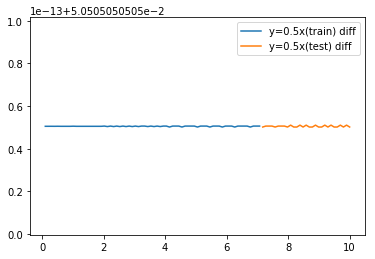Difference range of test data is same as range of training data.
So it is suitable for prediction target.
And we add predicted difference to original y.
Then we get good prediction.

```# Train and Predict difference
reg = RandomForestRegressor(random_state=0)
reg = reg.fit(np.array(df_train["x"]).reshape(-1,1), df_train["y_diff"])
y_diff_pred = reg.predict(np.array(df_test["x"]).reshape(-1,1))

y_pred = []
for i in range(0,len(df_test["x"])):
if i == 0:
y_pred.append(df_train["y"].iloc[-1] + y_diff_pred[i])
else:
y_pred.append(y_pred[i-1]+y_diff_pred[i])

print(y_pred)

# Show graph
plt.plot(df_train["x"], df_train["y"], label="y=0.5x(train)")
plt.plot(df_test["x"], df_test["y"], label="y=0.5x(test)")
plt.plot(df_test["x"], y_pred, label="y=0.5x(pred)")
plt.legend()
plt.show()
```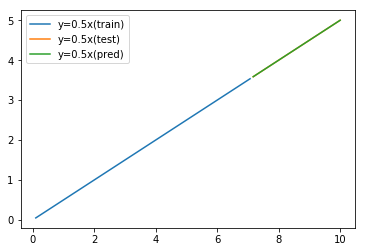## Finally

• Flat prediction result is due to Decision tree architecture.
• Predictable range of Decision tree depends on training data.
• With using difference or rate, you can predict unlimited range target.KRSW

Translate »